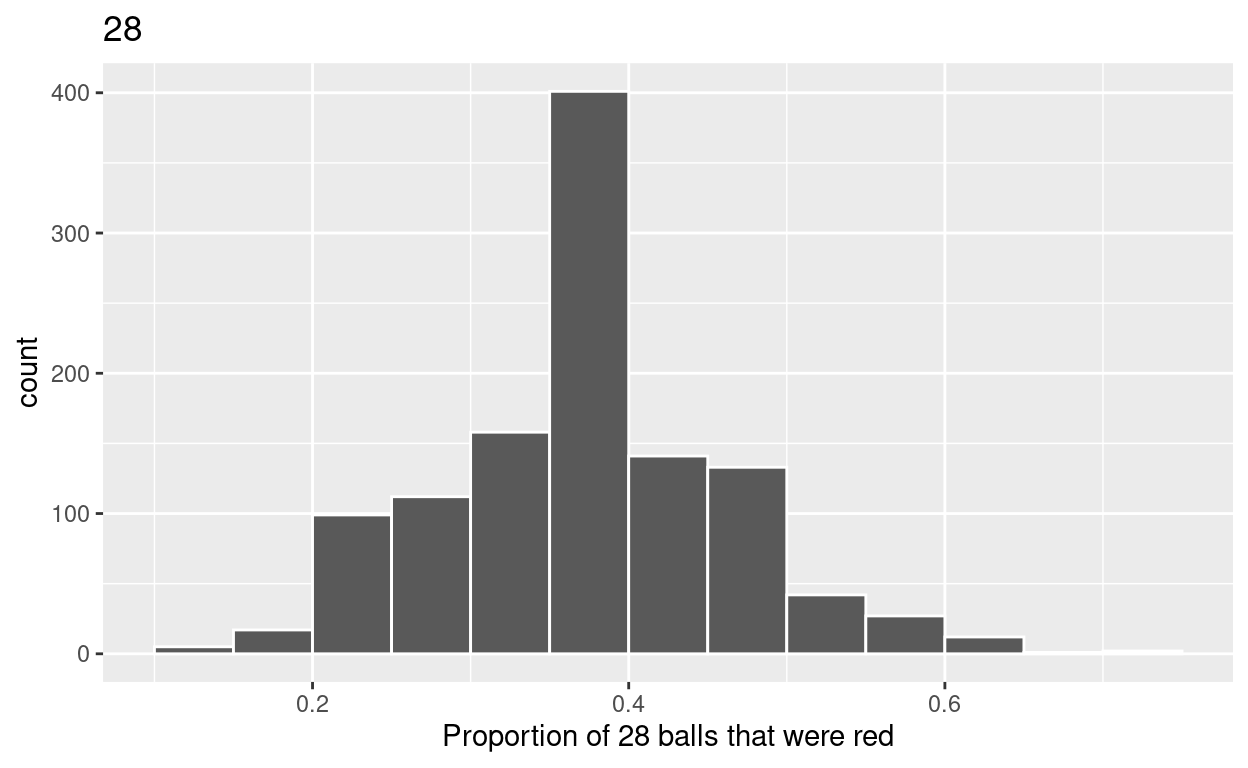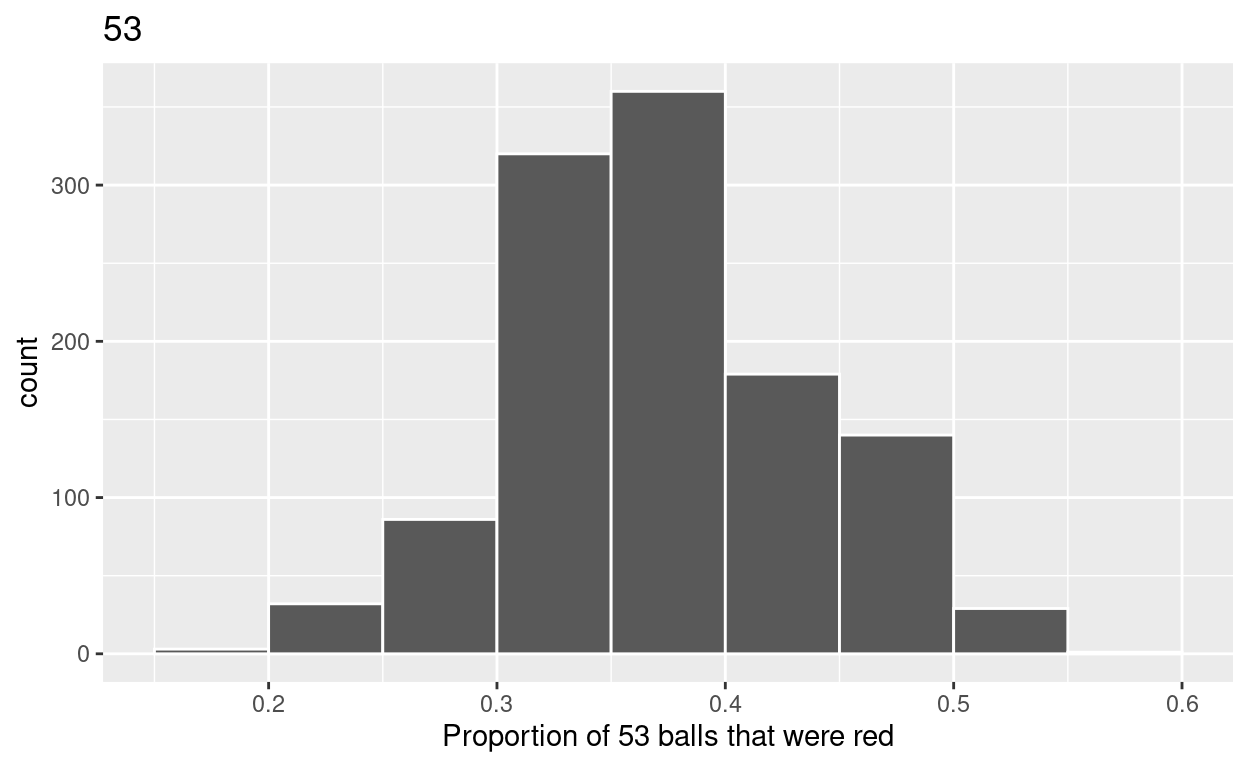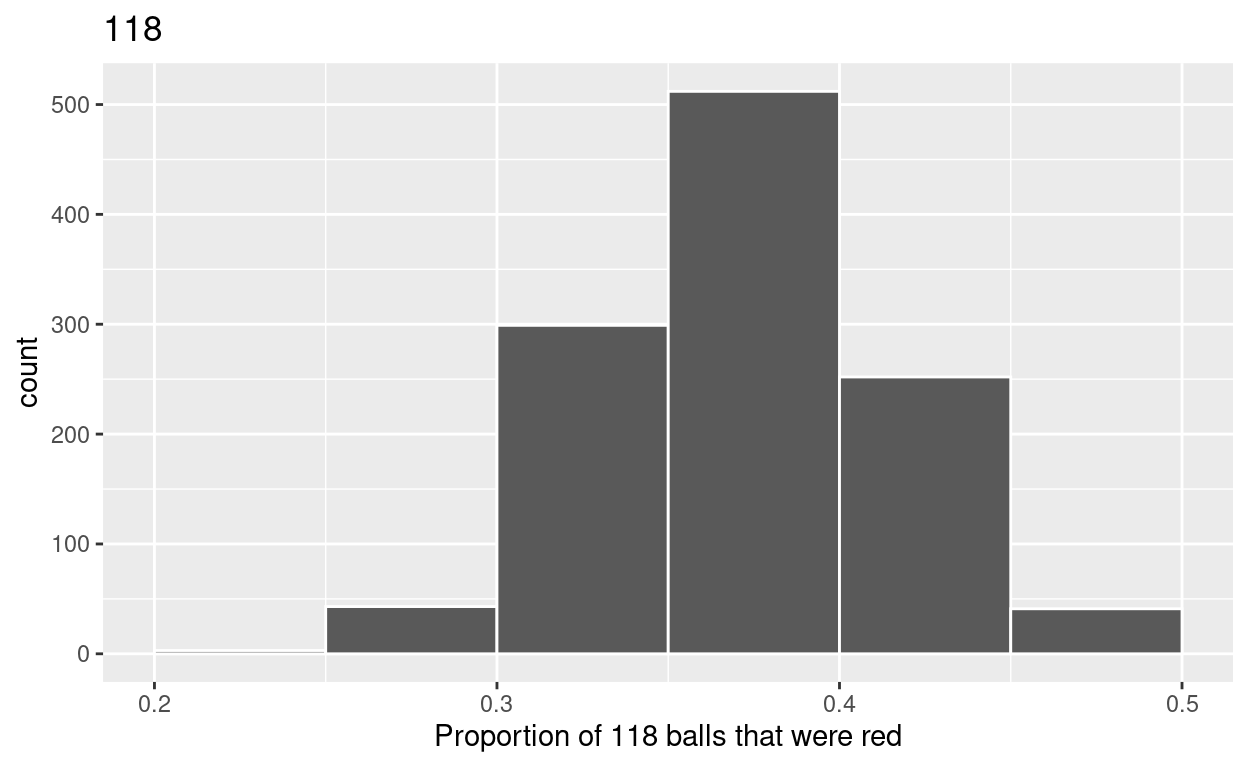# Sampling

Based on Chapter 7 of ModernDive. Code for Quiz 11.

1. Load the R package we will use.
``````library(tidyverse)
library(moderndive)
``````
1. Quiz questions
• Replace all the instances of ‘SEE QUIZ’. These are inputs from your moodle quiz.
• Replace all the instances of ‘???’. These are answers on your moodle quiz.
• Run all the individual code chunks to make sure the answers in this file correspond with your quiz answers
• After you check all your code chunks run then you can knit it. It won’t knit until the ??? are replaced
• The quiz assumes that you have watched the videos and worked through the examples in Chapter 7 of ModernDive

# Question:

7.2.4 in Modern Dive with different sample sizes and repetitions

• Make sure you have installed and loaded the `tidyverse` and the `moderndive` packages
• Fill in the blanks
• Put the command you use in the Rchunks in your Rmd file for this quiz.

Modify the code for comparing differnet sample sizes from the virtual bowl

Segment 1: sample size = 28

1. Take 1150 samples of size of 28 instead of 1000 replicates of size 25 from the `bowl` dataset. Assign the output to virtual_samples_28
``````virtual_samples_28  <- bowl  %>%
rep_sample_n(size = 28, reps = 1150)
``````
1. Compute resulting 1150 replicates of proportion red
• group_by replicate THEN
• create variable red equal to the sum of all the red balls
• create variable prop_red equal to variable red / 28
• Assign the output to virtual_prop_red_28
``````virtual_prop_red_28 <- virtual_samples_28 %>%
group_by(replicate) %>%
summarise(red = sum(color == "red")) %>%
mutate(prop_red = red / 28)
``````
1. Plot distribution of virtual_prop_red_28 via a histogram
• use labs to
• label x axis = “Proportion of 28 balls that were red”
• create title = “28”
``````ggplot(virtual_prop_red_28, aes(x = prop_red)) +
geom_histogram(binwidth = 0.05, boundary = 0.4, color = "white") +
labs(x = "Proportion of 28 balls that were red", title = "28")
``````Segment 2: sample size = 53

1. Take 1150 samples of size of 53 instead of 1000 replicates of size 50. Assign the output to virtual_samples_53
``````virtual_samples_53  <- bowl  %>%
rep_sample_n(size = 53, reps = 1150)
``````
1. Compute resulting 1150 replicates of proportion red
• group_by replicate THEN
• create variable red equal to the sum of all the red balls
• create variable prop_red equal to variable red / 53
• Assign the output to virtual_prop_red_53
``````virtual_prop_red_53 <- virtual_samples_53 %>%
group_by(replicate) %>%
summarise(red = sum(color == "red")) %>%
mutate(prop_red = red / 53)
``````
1. Plot distribution of virtual_prop_red_53 via a histogram
• use labs to
• label x axis = “Proportion of 53 balls that were red”
• create title = “53”
``````ggplot(virtual_prop_red_53, aes(x = prop_red)) +
geom_histogram(binwidth = 0.05, boundary = 0.4, color = "white") +
labs(x = "Proportion of 53 balls that were red", title = "53")
``````Segment 3: sample size = 118

1. Take 1150 samples of size of 118 instead of 1000 replicates of size 50. Assign the output to virtual_samples_118
``````virtual_samples_118  <- bowl  %>%
rep_sample_n(size = 118, reps = 1150)
``````
1. Compute resulting 1150 replicates of proportion red
• group_by replicate THEN
• create variable red equal to the sum of all the red balls
• create variable prop_red equal to variable red / 118
• Assign the output to virtual_prop_red_118
``````virtual_prop_red_118 <- virtual_samples_118 %>%
group_by(replicate) %>%
summarise(red = sum(color == "red")) %>%
mutate(prop_red = red / 118)
``````
1. Plot distribution of virtual_prop_red_118 via a histogram
• use labs to
• label x axis = “Proportion of 118 balls that were red”
• create title = “118”
``````ggplot(virtual_prop_red_118, aes(x = prop_red)) +
geom_histogram(binwidth = 0.05, boundary = 0.4, color = "white") +
labs(x = "Proportion of 118 balls that were red", title = "118")
``````Calculate the standard deviations for your three sets of SEE QUIZ values of `prop_red` using the `standard deviation`

n = 28

``````virtual_prop_red_28  %>%
summarize(sd = sd(prop_red))
``````
``````# A tibble: 1 × 1
sd
<dbl>
1 0.0903``````

n = 53

``````virtual_prop_red_53  %>%
summarize(sd = sd(prop_red))
``````
``````# A tibble: 1 × 1
sd
<dbl>
1 0.0648``````

n = 118

``````virtual_prop_red_118  %>%
summarize(sd = sd(prop_red))
``````
``````# A tibble: 1 × 1
sd
<dbl>
1 0.0429``````

The distribution with sample size, n = 118, has the smallest standard deviation (spread) around the estimated proportion of red balls.

``````ggsave(filename = "preview.png",
path = here::here("_posts", "2022-04-19-sampling"))
``````